### Home > CALC > Chapter 11 > Lesson 11.1.2 > Problem11-20

11-20.
1. Consider the infinite series below. For each, decide if it converges or diverges and justify your conclusion. State the test you used. Homework Help ✎

1.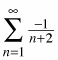2.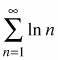3.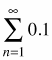4.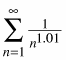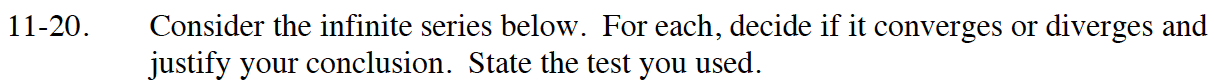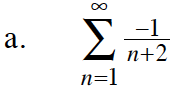This is a variation of the harmonic series.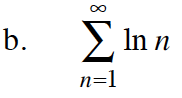$\text{What is }\lim_{n\to\infty}\ln(n)?$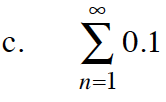$\text{What is }\lim_{n\to\infty}0.1?$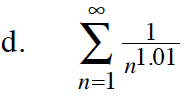This is a p-series.